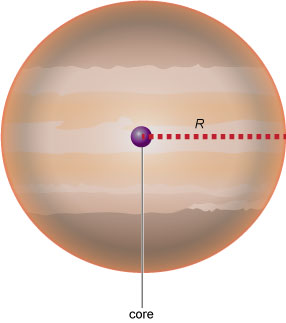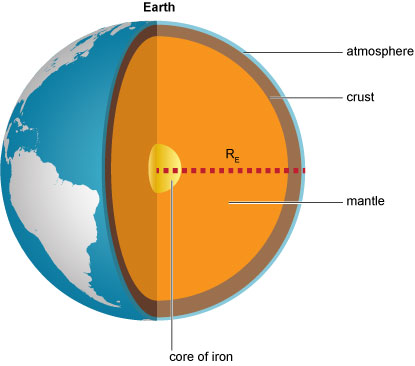An introduction to exoplanets

Start this free course now. Just create an account and sign in. Enrol and complete the course for a free statement of participation or digital badge if available.

Free course

# 4.1  Planet sizes

Earlier this week, you learned that the radius is used as a measurement of size in planets. Generally, if people need to calculate something that depends on a radius, they use the letter r to stand for the radius. For the purpose of this course, we will use a capital R for a planet’s radius (Figure 6) and a lower case r for other radii. The radius of the Earth is 6378 km.Figure 6  The radius, R, of a planet is the distance from the centre to the surface

When astronomers discuss the sizes of planets, it is cumbersome to use kilometres. It is far more convenient to introduce the unit 1 Earth radius, written 1 RE (or sometimes 1 R), when discussing terrestrial planets (Figure 7). It is obvious that a planet with a radius of say 1.1 RE is slightly bigger than Earth, 10 per cent bigger to be exact. On the other hand, if a planet had a radius of 7020 km, you probably wouldn’t immediately know how much bigger or smaller than Earth it is.Figure 7  The radius of the Earth, 1 RE, is used as a unit to measure the sizes of terrestrial planets

Similarly, when discussing giant planets, it is most convenient to use Jupiter’s radius, so giant planet radii are generally given in units of RJ.

EXO_1